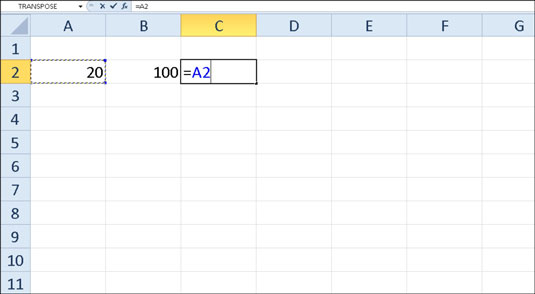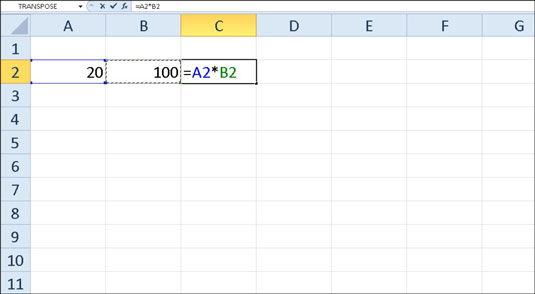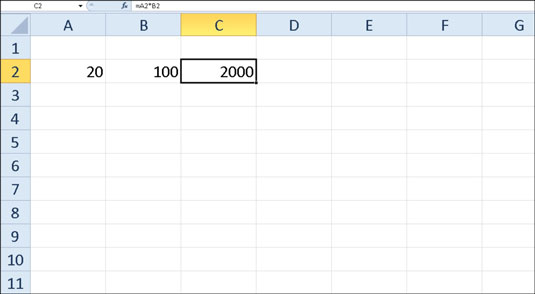##### Excel 2010 For DummiesFormulas are the real workhorses of an Excel 2010 worksheet. If you set up a formula properly, it computes the correct answer when you enter it into a cell. You can set up a formula from the functions excel provides or create your own custom excel function. From then on, it keeps itself up to date, recalculating the results whenever you change any of the values that the formula uses.

You let Excel know that you're about to enter a formula in the current cell by entering the equal sign (=). Some formulas follow the equal sign with a built-in function such as SUM or AVERAGE. Many simple formulas use a series of values or cell references that contain values separated by one or more of the following mathematical operators:

This Mathematical Operator . . . . . . Is Used For
- (minus sign or hyphen) Subtraction
* (asterisk) Multiplication
/ (slash) Division
^ (caret) Raising a number to an exponential power
For example, to create a formula in cell C2 that multiplies a value entered in cell A2 by a value in cell B2, enter the following formula in cell C2: =A2*B2

To enter this formula in cell C2, follow these steps:

1. Select cell C2.

2. Type the entire formula =A2*B2 in the cell.

3. Press Enter.

Or
1. Select cell C2.

2. Type = (equal sign).

3. Select cell A2 in the worksheet by using the mouse or the keyboard.

This action places the cell reference A2 in the formula in the cell.To start the formula, type =, and then select cell A2.
4. Type * (Shift+8 on the top row of the keyboard).

5. Select cell B2 in the worksheet by using the mouse or the keyboard.

This action places the cell reference B2 in the formula.6. Press Enter.

Excel displays the calculated answer in cell C2 and the formula =A2*B2 in the Formula bar.If you select the cell you want to use in a formula, either by clicking it or by moving the cell cursor to it, you have less chance of entering the wrong cell reference.

Now comes the fun part: After creating a formula that refers to the values in certain cells (rather than containing those values itself), you can change the values in those cells, and Excel automatically recalculates the formula, using these new values and displaying the updated answer in the worksheet. Using the example shown in the figures, suppose that you change the value in cell B2 from 100 to 50. The moment that you complete this change in cell B2, Excel recalculates the formula and displays the new answer, 1000, in cell C2.

Did this glimpse into Excel formulas leave you longing for more information and insight about Microsoft's popular spreadsheet program? You're free to test drive any of the For Dummies eLearning courses. Pick your course (you may be interested in more from Excel 2010), fill out a quick registration, and then give eLearning a spin with the Try It! button. You'll be right on course for more trusted know how: The full version's also available at Excel 2010.